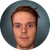# Merge two arrays

400
3
08-07-2019 07:30 AMOccasional Contributor

Hi all

Am struggling to merge two result arrays of species that I wish to display as a single list with no duplicates.

The resulting arrays are in this format:

0: {species_common: "Black Kite"}

1: {species_common: "White Stork"}

How would I go about merging both lists so I can display just the values like Black Kite, White Stork, etc in one list?

I have tried doing this

const object1 = [spcAtObsCommon];
const object2 = [spcAtISitesCommon];

const object3 = {...object1, ...object2 };

if I do a console.log(object3) all I see is the results from object2 in exactly the same format. What am I doing wrong? Any advice or pointers would be gratefully received.

Best,

Mark

1 Solution

Accepted SolutionsOccasional Contributor

Many thanks for the responses, after some reading around the subject I ended up using the following method..

newArray1 = spcAtObsCommon.map(object => object.species_common);
newArray2 = spcAtISitesCommon.map(object => object.species_common);

console.log([...new Set([...newArray1, ...newArray2])]);

3 RepliesbyEsri Contributor

This may work:

``````let set = new Set();

Object.keys(spcAtISitesCommon).forEach((key)=> {
});

Object.keys(spcAtObsCommon).forEach((key)=> {
});‍‍‍‍‍‍‍‍‍‍‍‍‍‍‍‍‍‍‍‍‍‍‍‍‍‍‍‍‍‍‍‍‍‍‍‍‍‍‍‍‍‍‍‍‍‍‍‍‍‍‍‍‍‍‍‍‍‍‍‍‍‍‍‍‍‍‍‍‍‍‍‍‍‍‍‍‍‍‍‍‍``````

A set is essentially a unique array. We are iterating through the objects and adding each species to the set.

Edit: my understanding being that the data is structured like:

``````const spcAtObsCommon= {
0: {species_common: "Black Kite"},
1: {species_common: "White Stork"}
}‍‍‍‍‍‍‍‍‍‍‍‍‍‍‍‍‍‍‍‍``````byEsri Regular Contributor

Hi Mark,

it could be based on which js version you are using and which browser you are trying to support as well.

To just merge the arrays (without removing duplicates)

### ES5 version use`Array.concat`:

``var array1 = ["Vijendra","Singh"];var array2 = ["Singh", "Shakya"];var array3 = array1.concat(array2); // Merges both arrays// [ 'Vijendra', 'Singh', 'Singh', 'Shakya' ]``

### ES6 version usedestructuring

``const array1 = ["Vijendra","Singh"];const array2 = ["Singh", "Shakya"];const array3 = [...array1, ...array2];``

Since there is no 'built in' way to remove duplicates (ECMA-262 actually has `Array.forEach` which would be great for this), we have to do it manually:

``Array.prototype.unique = function() {    var a = this.concat();    for(var i=0; i<a.length; ++i) {        for(var j=i+1; j<a.length; ++j) {            if(a[i] === a[j])                a.splice(j--, 1);        }    }     return a;};``

Then, to use it:

``var array1 = ["Vijendra","Singh"];var array2 = ["Singh", "Shakya"];// Merges both arrays and gets unique itemsvar array3 = array1.concat(array2).unique(); ``

This will also preserve the order of the arrays (i.e, no sorting needed).

Since many people are annoyed about prototype augmentation of `Array.prototype` and `for in`loops, here is a less invasive way to use it:

``function arrayUnique(array) {    var a = array.concat();    for(var i=0; i<a.length; ++i) {        for(var j=i+1; j<a.length; ++j) {            if(a[i] === a[j])                a.splice(j--, 1);        }    }     return a;}var array1 = ["Vijendra","Singh"];var array2 = ["Singh", "Shakya"];    // Merges both arrays and gets unique itemsvar array3 = arrayUnique(array1.concat(array2));``

For those who are fortunate enough to work with browsers where ES5 is available, you can use `Object.defineProperty` like this:

``Object.defineProperty(Array.prototype, 'unique', {    enumerable: false,    configurable: false,    writable: false,    value: function() {        var a = this.concat();        for(var i=0; i<a.length; ++i) {            for(var j=i+1; j<a.length; ++j) {                if(a[i] === a[j])                    a.splice(j--, 1);            }        }         return a;    }});``

Below is the list for your reference.Occasional Contributor

Many thanks for the responses, after some reading around the subject I ended up using the following method..

newArray1 = spcAtObsCommon.map(object => object.species_common);
newArray2 = spcAtISitesCommon.map(object => object.species_common);

console.log([...new Set([...newArray1, ...newArray2])]);